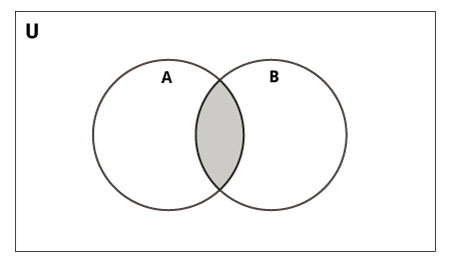# Intersection() function Python

In this article, we will learn about intersection() function that can be performed on any given set. According to mathematics intersection means finding out common elements from the two sets.## Syntax

<set name>.intersection(<set a1> <set a2> ……..)

## Return Value

common elements in sets passed as arguments.

## Example

set_1 = {'t','u','t','o','r','i','a','l'}
set_2 = {'p','o','i','n','t'}
set_3 = {'t','u','t'}
#intersection of two sets
print("set1 intersection set2 : ", set_1.intersection(set_2))
# intersection of three sets
print("set1 intersection set2 intersection set3 :", set_1.intersection(set_2,set_3))

## Output

set1 intersection set2 : {'i', 'o', 't'}
set1 intersection set2 intersection set3 : {'t'}

## Explanation

Here a search is made to find the common elements by interpreter implicitly and is returned back as a set to the respective reference.

## Conclusion

In this article, we learned about the implementation and usage of the intersection function present in Python 3.x. Or earlier.